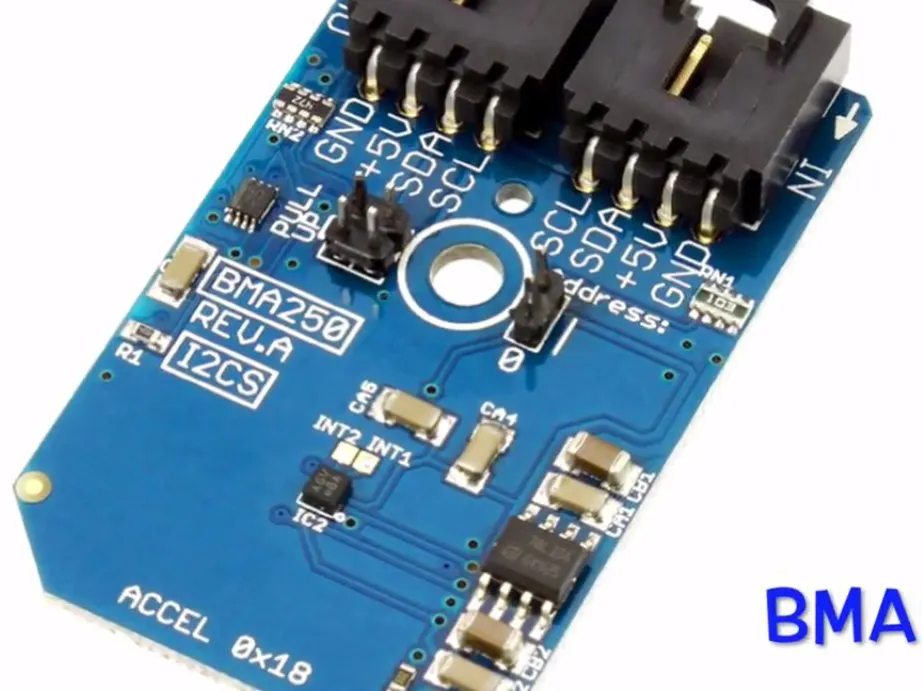Acceleration Measurement Using BMA250 and Arduino Nano

BMA250 is a small, thin, ultralow power, 3-axis accelerometer with high resolution (13-bit) measurement at up to ±16 g.

• 2,071 views
• 1 comment
• 1 respect

Components and suppliesArduino Nano R3
×1
 BMA250 Digital Triaxial ±2g to ±16g Acceleration Sensor with Intelligent On-Chip Motion-Triggered Interrupt Controller I²C Mini Module
×1
 I²C Cable 6-inch
×1
 ControlEverything.com I2C Shield for Arduino Nano
×1

Introduction

BMA250 is a small, thin, ultralow power, 3-axis accelerometer with high resolution (13-bit) measurement at up to ±16 g. Digital output data is formatted as 16-bit twos complement and is accessible through I2C digital interface. It measures the static acceleration of gravity in tilt-sensing applications, as well as dynamic acceleration resulting from motion or shock. Its high resolution (3.9 mg/LSB) enables measurement of inclination changes less than 1.0°.

In this tutorial we are going to measure the acceleration in all the three perpendicular axes using BMA250 and Arduino Nano.

Step 1: Hardware Required:

The materials that we need for accomplishing our goal includes the following hardware components:

1. BMA250

2. Arduino Nano

Step 2: Hardware Hookup:

The hardware hookup section basically explains the wiring connections required between the sensor and the Arduino. Ensuring correct connections is the basic necessity while working on any system for the desired output. So, the requisite connections are as follows:

• The BMA250 will work over I2C. Here is the example wiring diagram, demonstrating how to wire up each interface of the sensor.
• Out-of-the-box, the board is configured for an I2C interface, as such we recommend using this hookup if you’re otherwise agnostic. All you need is four wires!

Only four connections are required Vcc, Gnd, SCL and SDA pins and these are connected with the help of I2C cable. These connections are demonstrated in the pictures above.

Step 3: Arduino Code For Acceleration Measurement:

Lets start with the Arduino code now.

While using the sensor module with the Arduino, we include Wire.h library. "Wire" library contains the functions which facilitate the I2C communication between the sensor and the Arduino board.

The entire Arduino code is given below for the convenience of the user:

#include
// BMA250 I2C address is 0x18(24)
void setup()
{
// Initialise I2C communication as MASTER
Wire.begin();
// Initialise Serial Communication, set baud rate = 9600
Serial.begin(9600);
// Start I2C Transmission
// Select range selection register
Wire.write(0x0F);
// Set range +/- 2g
Wire.write(0x03);
// Stop I2C Transmission
Wire.endTransmission();
// Start I2C Transmission
// Select bandwidth register
Wire.write(0x10);
// Set bandwidth 7.81 Hz
Wire.write(0x08);
// Stop I2C Transmission
Wire.endTransmission();  delay(300);}
void loop()
{
unsigned int data;
// Start I2C Transmission
// Select Data Registers (0x02 − 0x07)
Wire.write(0x02);
// Stop I2C Transmission
Wire.endTransmission();
// Request 6 bytes
// Read the six bytes
// xAccl lsb, xAccl msb, yAccl lsb, yAccl msb, zAccl lsb, zAccl msb
if(Wire.available() == 6)
{
}
delay(300);
// Convert the data to 10 bits
float xAccl = ((data * 256.0) + (data & 0xC0)) / 64;
if (xAccl > 511)
{
xAccl -= 1024;
}
float yAccl = ((data * 256.0) + (data & 0xC0)) / 64;
if (yAccl > 511)
{
yAccl -= 1024;
}
float zAccl = ((data * 256.0) + (data & 0xC0)) / 64;
if (zAccl > 511)
{
zAccl -= 1024;
}
// Output data to the serial monitor
Serial.print("Acceleration in X-Axis :");
Serial.println(xAccl);
Serial.print("Acceleration in Y-Axis :");
Serial.println(yAccl);
Serial.print("Acceleration in Z-Axis :");
Serial.println(zAccl) ;
}

In wire library Wire.write() and Wire.read() is used to write the commands and read the sensor output. Serial.print() and Serial.println() are used to display the sensor output on the serial monitor of the Arduino IDE.

The sensor output is shown in the picture above.

Step 4: Applications:

Accelerometers like BMA250 mostly find its application in the games and display profile switching. This sensor module is also employed in the advanced power management system for mobile applications. BMA250 is a triaxial digital acceleration sensor which is incorporated with an intelligent on-chip motion triggered interrupt controller.

Published on

June 27, 2016
See similar projects
you might like

• 3,293 views
• 5 respects

• 1,391 views
• 1 comment
• 0 respects

• 3,137 views
• 1 respect

• 1,278 views